Name:    3rd Grade Basic Math Test 5

Multiple Choice
Identify the choice that best completes the statement or answers the question.

1.

Measurement – Area, Perimeter, Circumference – RIT 171 – 180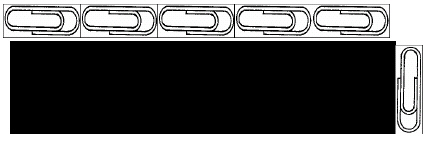About how many paper clips would it take to go around this shape?
 a. 7 d. 14 b. 10 e. 12 c. 4

2.

Find the area in square units.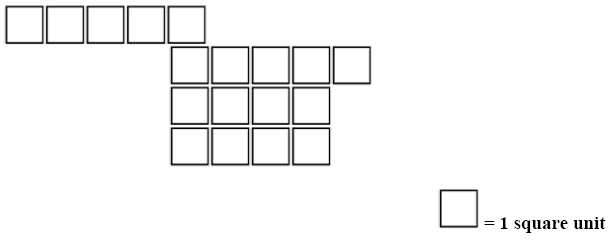a. 18 d. 12 b. 16 e. 24 c. 20

3.

Measurement – Length, Height, Volume – RIT 171 – 180

You can find these containers around your house. Which one would hold the most water?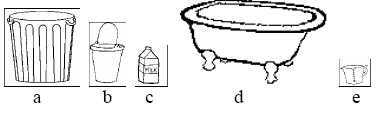a. e d. d b. c e. b c. a

4.

How long is the lightbulb?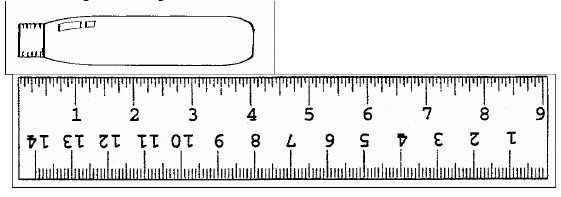a. 4 inches d. 1 inch b. 3 inches e. 5 inches c. 2 inches

5.

How much do the potatoes weigh?a. 9 d. 0 b. 6 e. 4 c. 3

6.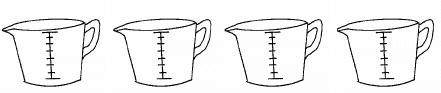These 4 cups are equal to ______?
 a. 1 pint d. 2 quarts b. 1 gallon e. 3 pints c. 1 quart

7.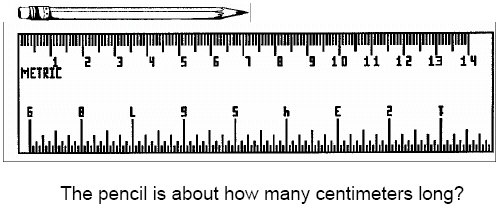a. 5 cm d. 4 cm b. 6 cm e. 8 cm c. 7 cm

8.

Measurement – Money – RIT 171 – 180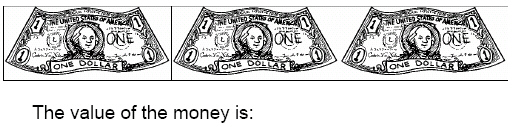a. \$2.00 d. \$4.00 b. \$1.00 e. \$30.00 c. \$3.00

9.

Which collection of coins is worth 63?
 a.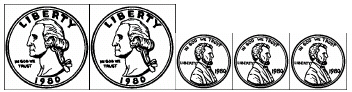b.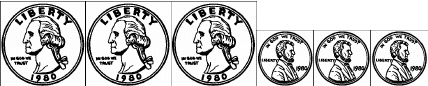c.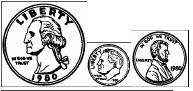d.e.10.

Measurement – Time, Temperature – RIT 171 – 180a. 3:30 d. 5:15 b. 6:45 e. 3:06 c. 6:15

11.

What time is it?a. 10:08 d. 1:10 b. 10:02 e. 11:08 c. 1:52

12.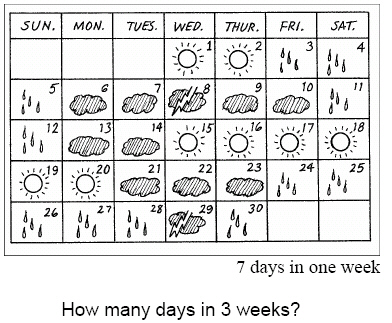a. 10 d. 4 b. 21 e. 3 c. 7

13.

Measurement – Area, Perimeter, Circumference – RIT 181 - 190a. 10 d. 12 b. 11 e. 8 c. 9

14.a. 18 square units d. 16 square units b. 9 square units e. 5 square units c. 20 square units

15.

What is the perimeter?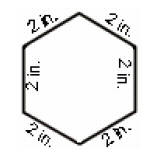a. 2 inches d. 12 inches b. 8 inches e. 6 inches c. 10 inches

16.

Measurement – Length, Height, Volume – RIT 181 – 190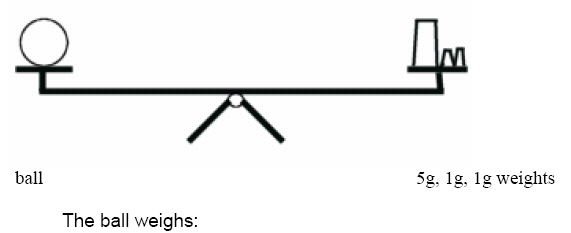a. 5g d. 6g b. 2g e. 7g c. 1g

17.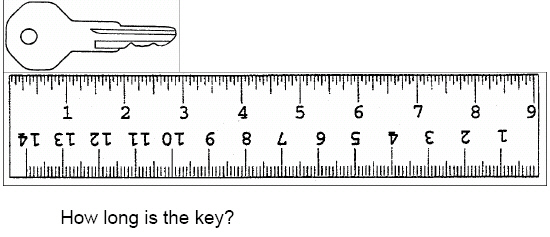a. 3 inches d. 5 inches b. 4 inches e. 1 inch c. 2 inches

18.

Measurement – Money – RIT 181 – 190

Which collection of coins has pennies?
 a.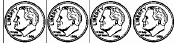b.c.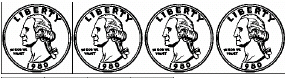d.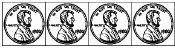e.19.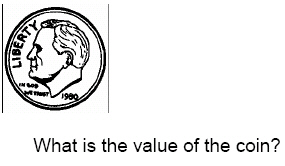a. 1¢ d. 50¢ b. 5¢ e. 10¢ c. 25¢

20.

3 dollars, 1 quarter, and 1 dime equals:
 a. \$3.35 d. \$3.25 b. \$1.35 e. \$3.10 c. \$2.35

21.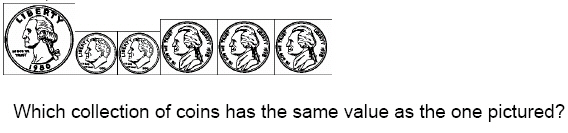a.b.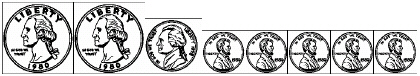c.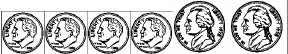d.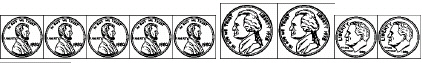e.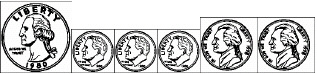22.

A candy bar cost 35. Mary gave the clerk \$1.00. Which shows the correct
change given to him.
 a.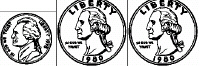d.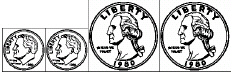b.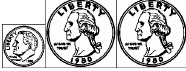e.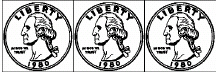c.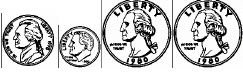23.

\$7.83
-\$4.95
 a. \$3.12 d. \$2.88 b. \$3.88 e. \$2.28 c. \$12.78